##### Basic Math & Pre-Algebra For Dummies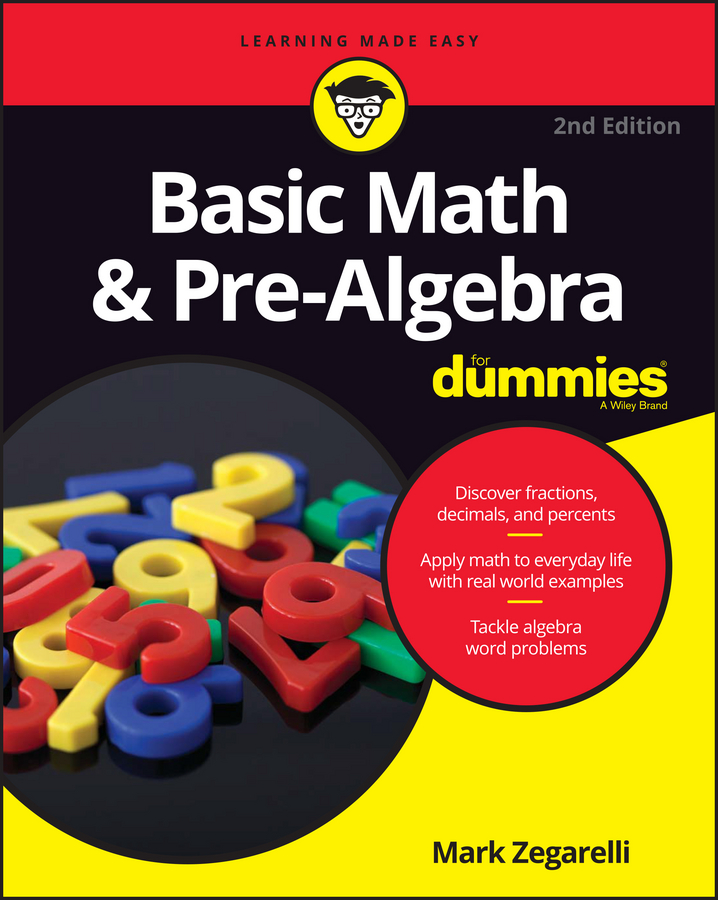You can use the number line to divide. For example, suppose you want to divide 6 by some other number. First, draw a number line that begins at 0 and ends at 6, as in the following figure.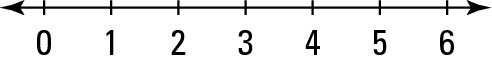Now, to find the answer to 6 ÷ 2, just split this number line into two equal parts, as shown in the following figure. This split (or division) occurs at 3, showing you that 6 ÷ 2 = 3.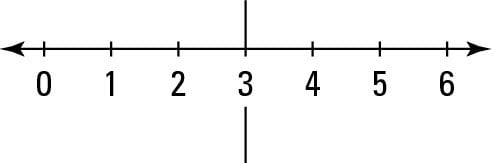Similarly, to divide 6 by 3, split the same number line into three equal parts, as in the following figure. This time you have two splits, so use the one closest to 0. This number line shows you that 6 ÷ 3 = 2.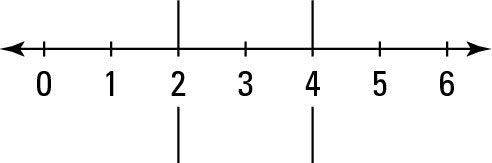But suppose you want to use the number line to divide a small number by a larger number. For example, maybe you want to know the answer to 3 ÷ 4. First draw a number line from 0 to 3. Then split it into four equal parts. Unfortunately, none of these splits has landed on a number. That’s not a mistake. You just have to add some new numbers to the number line, as you can see in the following figure.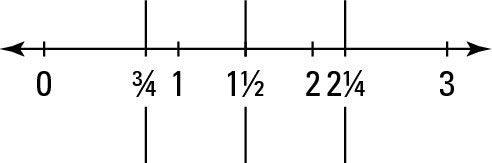Welcome to the world of fractions. With the number line labeled properly, you can see that the split closest to 0 is 3/4. This image tells you that 3 ÷ 4 = 3/4.

The similarity of the expression 3 ÷ 4 and the fraction 3/4 is no accident. Division and fractions are closely related. When you divide, you cut things up into equal parts, and fractions are often the result of this process.